# Algebra II : Polynomial Functions

## Example Questions

1 2 4 Next →

### Example Question #31 : Polynomial Functions

A baseball is thrown off the roof of a building 220 feet high at an initial upward speed of 72 feet per second; the height of the baseball relative to the ground is modeled by the function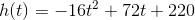How long does it take for the baseball to reach its highest point (nearest tenth of a second)?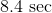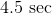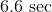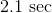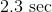Explanation:

The highest point of the ball is the vertex of the ball's parabolic path, so to find the number of secondsthat is takes to reach this point, it is necessary to find the vertex of the parabola of the graph of the functionThe parabola of the graph of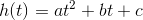has as its ordinate, or-coordinate,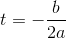,

so, setting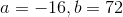,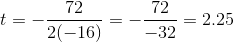,

which rounds to 2.3 seconds. This is the time that it takes the ball to reach the highest point of its path.

### Example Question #32 : Polynomial Functions

A baseball is thrown off the roof of a building 220 feet high at an initial upward speed of 72 feet per second; the height of the baseball relative to the ground is modeled by the functionHow long does it take for the baseball to hit the ground (nearest tenth of a second)?Explanation:

When the baseball hits the ground, its height is 0; therefore, we are looking forsuch that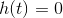,

or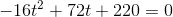This equation can most easily be solved using the quadratic formula. If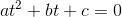,

then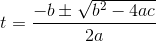Setting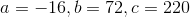: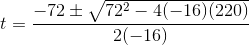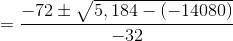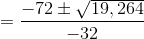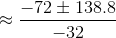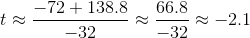We throw this out, since we cannot have "negative time".

The other is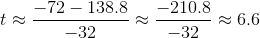This is positive, so we accept this answer. The ball hits the ground in about 6.6 seconds.

### Example Question #33 : Polynomial Functions

Find the product: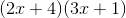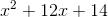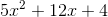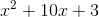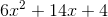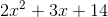Explanation:

Using the FOIL (first, outer, inner, last) method, you can expand the polynomial to get

first: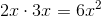outer: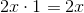inner: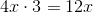lasts: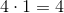From here, combine the like terms.### Example Question #34 : Polynomial Functions

What are the roots of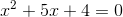?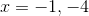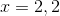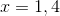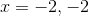Explanation:

In order to find the roots, we must factor the equation.

The factors of this equation are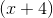and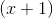.

Setting those two equal to zero, we get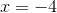and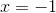.

1 2 4 Next →

### All Algebra II Resources